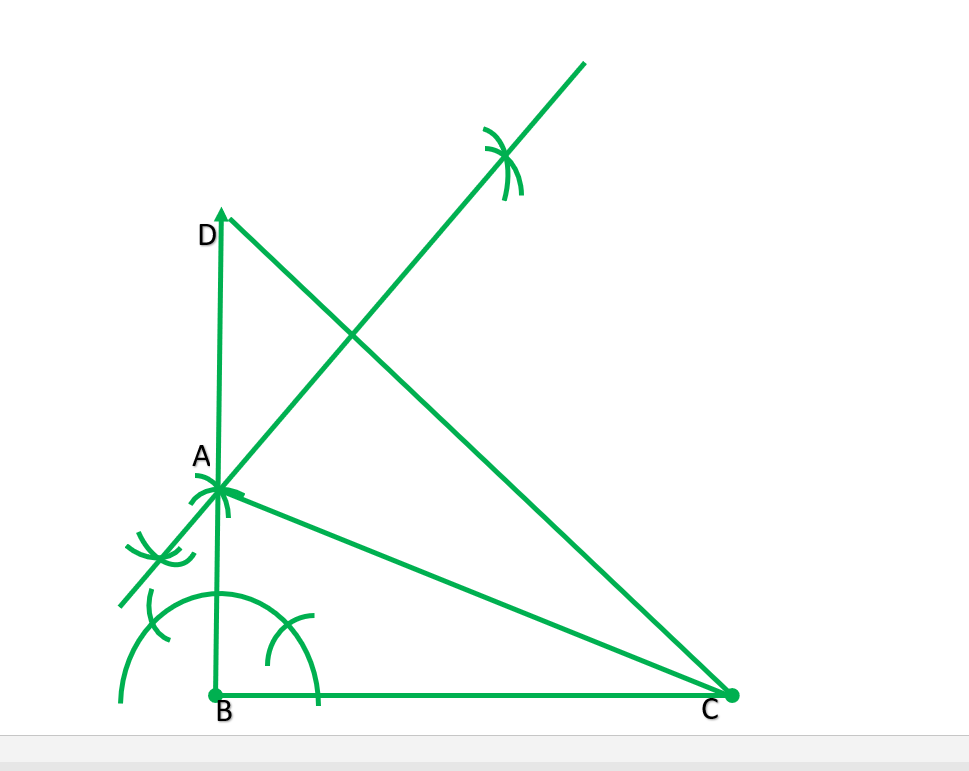Open In App
Related Articles
• NCERT Solutions for Class 9 Maths

# Class 9 NCERT Solutions- Chapter 11 Constructions – Exercise 11.2

### Question 1. Construct a ∆ ABC in which BC = 7 cm, ∠B = 75° and AB + AC = 13 cm.

Solution:

Steps of construction:

1. Draw a line segment BC base of cm is drawn.
2. At point B draw an angle of 75°.
3. Cut BD =13cm from BY.
4. Join ∠D which intersect BD at A.
5. Join AC. Now triangle ABC is the required triangle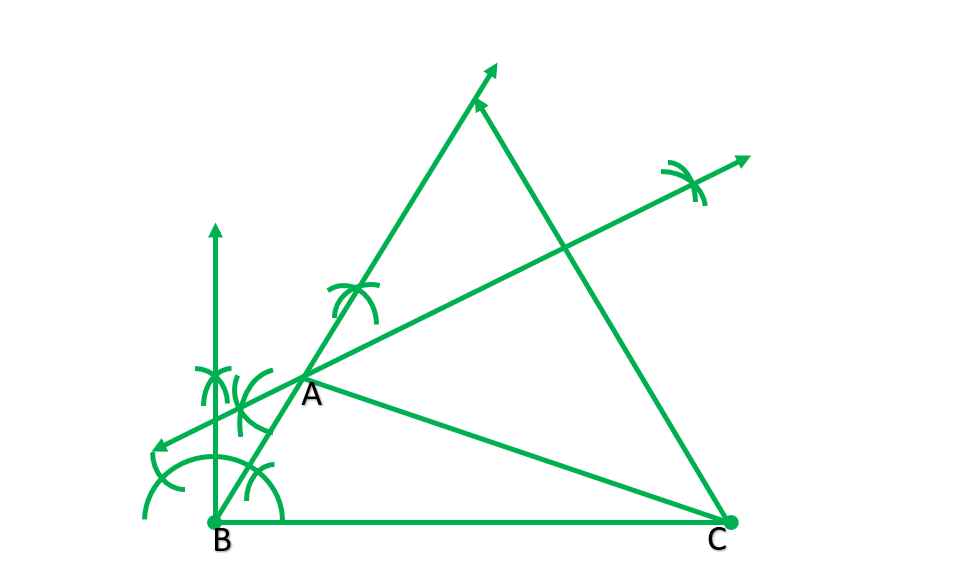### Question 2. Construct a ABC in which BC = 8 cm, ∠B = 45° and AB – AC = 35 cm.

Solution:

Steps of construction:

1. Draw  a line segment BC=8cm.
2. At point B, draw angle 45°.
3. Cut BD=3.5 from BY.
4. Join CD.
5. Draw perpendicular bisector of CD, which construct BY at A.
6. Join AC. NOW, ABC is the required triangle.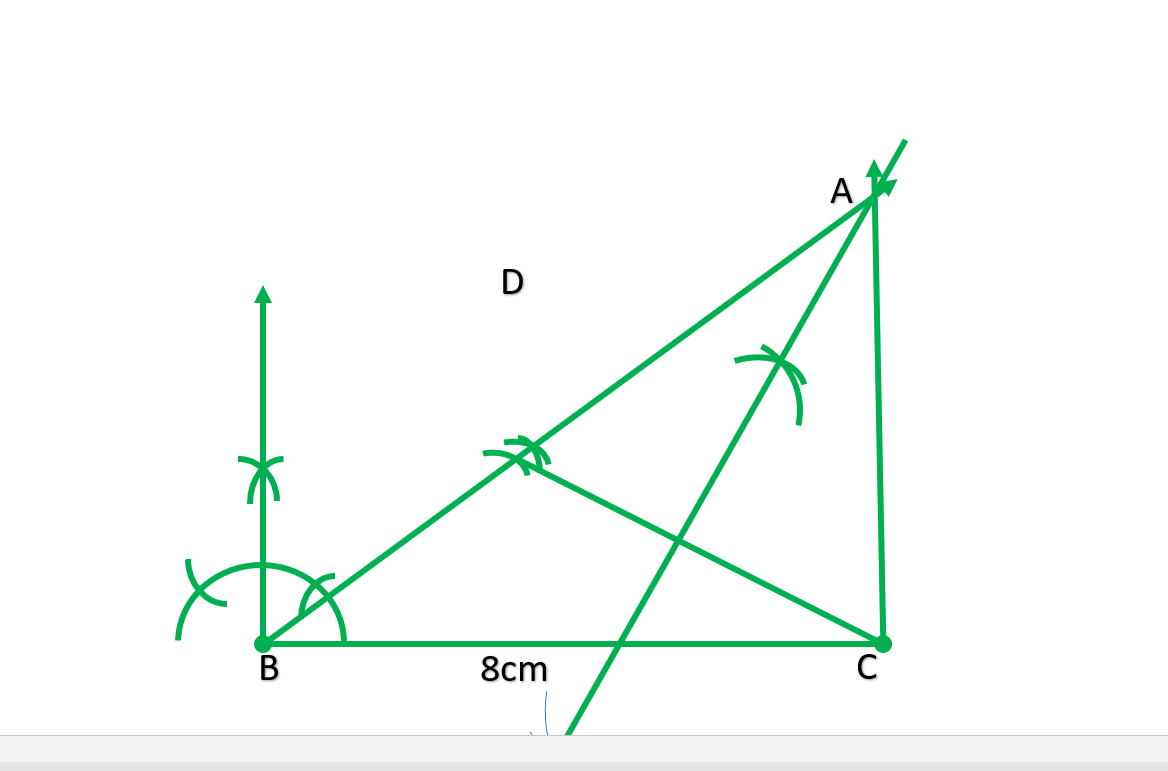### Question 3. Construct a ∆ ABC in which QR = 6 cm, ∠Q = 60° and PR – PQ = 2 cm.

Solution:

Steps of construction:

1. Draw a line segment QR=6cm.
2. At point Q draw angle 60°.
3. Extend PQ to Y’.
4. Cut QS =2cm from QY’.
5. Join RS.
6. Draw perpendicular bisector of RS which intersect QY at P.
7. Join PR. Now, PQR is the required triangle.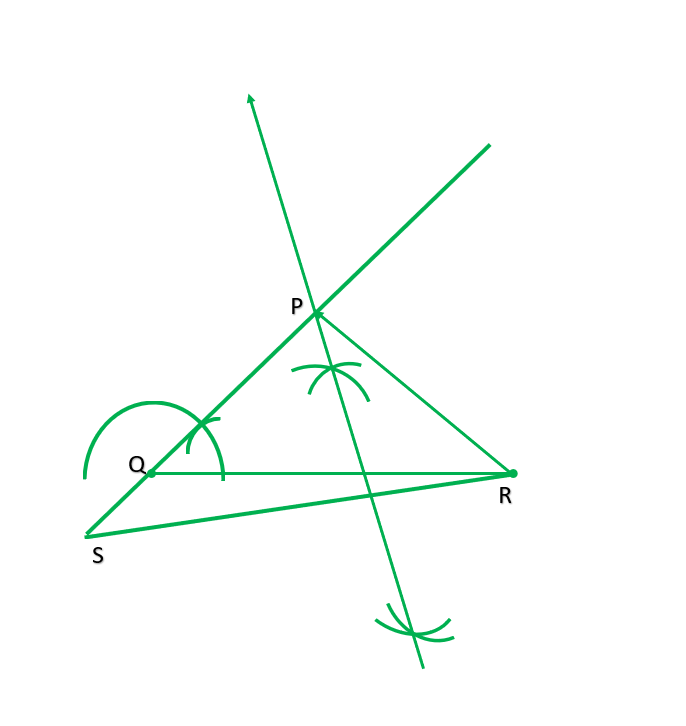### Question 4. Construct a ∆ XYZ in which ∠Y = 30°, ∠Y = 90° and XY + YZ + ZX = 11 cm.

Solution:

Steps of construction:

1. Draw a line segment AB=11cm.
2. At point A draw ∠BAP=30°.
3. At point B draw angle 90°.
4. Draw the bisector of ∠BAP and ∠ABR which intersect each other at X.
5. Join AX and BX.
6. Draw perpendicular bisector of AX and BX which intersect AB on Y and Z respectively.
7. Join XY and XZ. Then XYZ is the required triangle.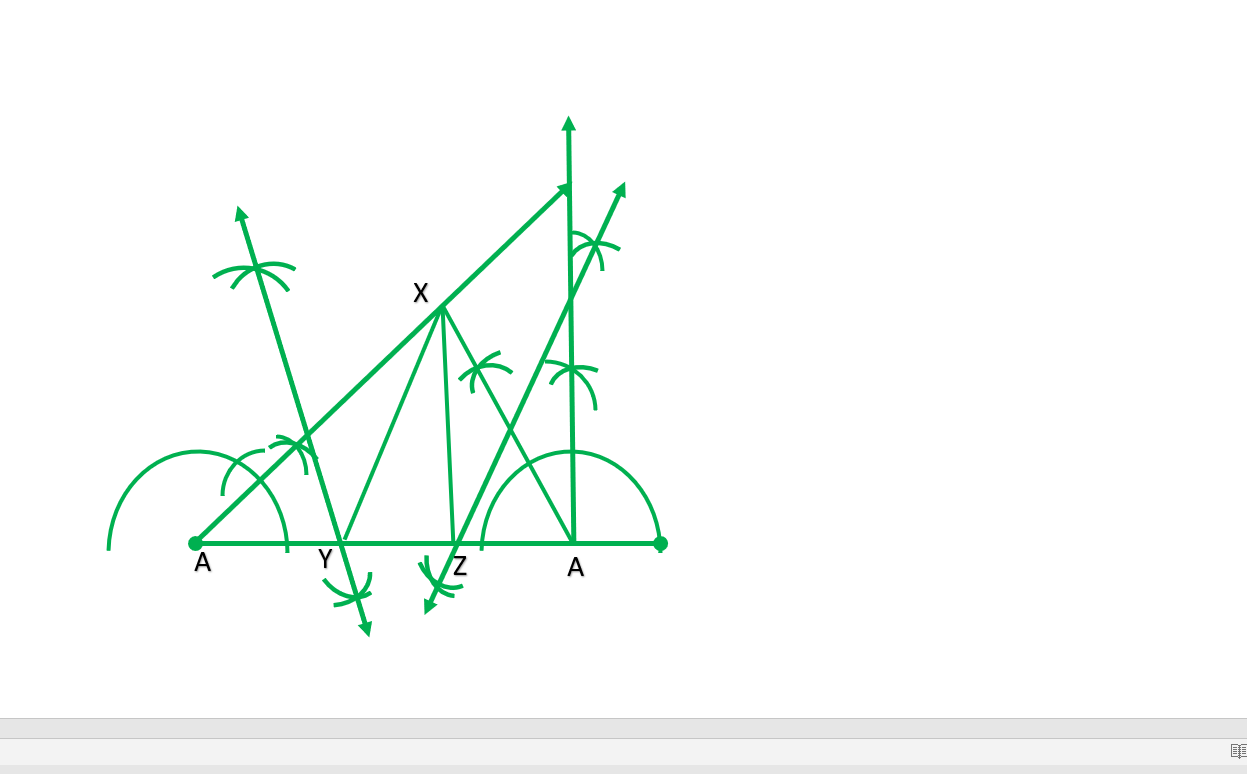### Question 5. Construct a right triangle whose base is 12 cm and sum of its hypotenuse and other side is 18 cm.

Solution:

Steps of construction:

1. Draw a line segment of BC=12cm.
2. At point B draw angle b=90°
3. Cut BD =18cm.
4. Join CD.
5. Draw perpendicular bisector of CD which intersect BD at point A.
6. Join AC. Now ABC is the required triangle.